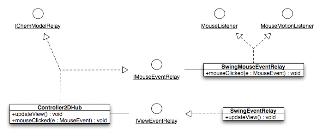Skip to main content

### Interface relays and controller modules

Sorry, but some more opaque UML diagram from the cdk.controller package. First, the Controller2DModules:Which is very simple, although the names are a bit clumsy. I would favour using a sub-package called "module" and then having "AddAtomModule", "ChangeFormalChargeModule", and so on. Doesn't really matter, as they will likely not be used very often.

The other image is more complex; it shows the class that implements IChemModelRelay and how it interacts with the two current Swing event handlers.The circles represent interfaces. The MouseListener and MouseMotionListener are, of course, the interfaces from the java.awt package that are normally used by java programs. Now to figure out how to actually use all this!

### How many isomers of C4H11N are there?

One of the most popular queries that lands people at this blog is about the isomers of C4H11N - which I suspect may be some kind of organic chemistry question on student homework. In any case, this post will describe how to find all members of a small space like this by hand rather than using software.

Firstly, lets connect all the hydrogens to the heavy atoms (C and N, in this case). For example:

Now eleven hydrogens can be distributed among these five heavy atoms in various ways. In fact this is the problem of partitioning a number into a list of other numbers which I've talked about before. These partitions and (possible) fragment lists are shown here:

One thing to notice is that all partitions have to have 5 parts - even if one of those parts is 0. That's not strictly a partition anymore, but never mind. The other important point is that some of the partitions lead to multiple fragment lists - [3, 3, 2, 2, 1] could have a CH+NH2 or an NH+CH2.

The final step is to connect u…

### The Gale-Ryser Theorem

This is a small aside. While reading a paper by Grüner, Laue, and Meringer on generation by homomorphism they mentioned the Gale-Ryser (GR) theorem. As it turns out, this is a nice small theorem closely related to the better known Erdős-Gallai (EG).

So, GR says that given two partitions of an integer (p and q) there exists a (0, 1) matrixA iff p*dominatesq such that the row sum vector r(A) = p and the column sum vector c(A) = q.

As with most mathematics, that's quite terse and full of terminology like 'dominates' : but it's relatively simple. Here is an example:

The partitions p and q are at the top left, they both sum to 10. Next, p is transposed to get p* = [5, 4, 1] and this is compared to q at the bottom left. Since the sum at each point in the sequence is greater (or equal) for p* than q, the former dominates. One possible matrix is at the top left with the row sum vector to the right, and the column sum vector below.

Finally, the matrix can be interpreted as a bi…

### Havel-Hakimi Algorithm for Generating Graphs from Degree Sequences

A degree sequence is an ordered list of degrees for the vertices of a graph. For example, here are some graphs and their degree sequences:

Clearly, each graph has only one degree sequence, but the reverse is not true - one degree sequence can correspond to many graphs. Finally, an ordered sequence of numbers (d1 >= d2 >= ... >= dn > 0) may not be the degree sequence of a graph - in other words, it is not graphical.

The Havel-Hakimi (HH) theorem gives us a way to test a degree sequence to see if it is graphical or not. As a side-effect, a graph is produced that realises the sequence. Note that it only produces one graph, not all of them. It proceeds by attaching the first vertex of highest degree to the next set of high-degree vertices. If there are none left to attach to, it has either used up all the sequence to produce a graph, or the sequence was not graphical.

The image above shows the HH algorithm at work on the sequence [3, 3, 2, 2, 1, 1]. Unfortunately, this produce…